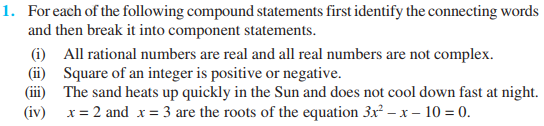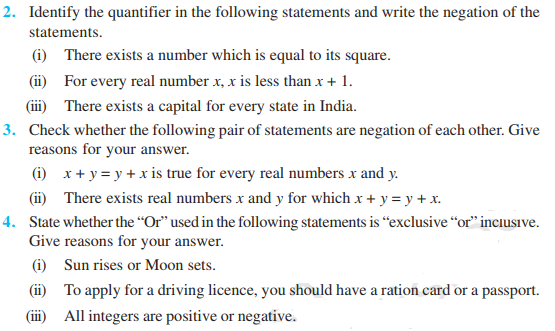# Mathematical Reasoning Class 11 Notes-Chapter 14

## What is Mathematical Reasoning?

In mathematical language, there are two kinds of reasoning – inductive and deductive Logic

Logic, on the other hand, is the study that is done with the method of reasoning. While proving the theorem, it provides us the rules for determining the validity of a given argument.

### What is Statement (Proposition)?

The basic unit involved in mathematical reasoning is a mathematical statement

A statement is an assertive sentence which is either true or false but not both a true statement is called a valid statement. Otherwise, it is called an invalid statement.

### Denotion of Statement

Statements are denoted by the small letters i.e., p, q, r … etc.

Example

• Mathematics is fun.
• This sentence is subjective in the sense that for those who like mathematics, it may be fun but for others, it may not be. This means that this sentence is not always true. Hence it is not a statement.
• A statement should be a “mathematically acceptable” statement
• An ambiguous sentence is not acceptable as a statement in mathematics.

### Methods to Check Validity

We can use various methods to check the validity of a statement such as:

• Methods using counterexample